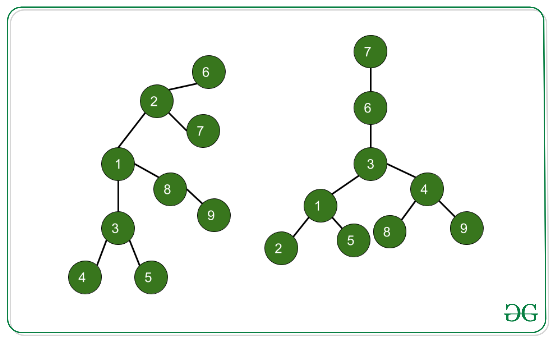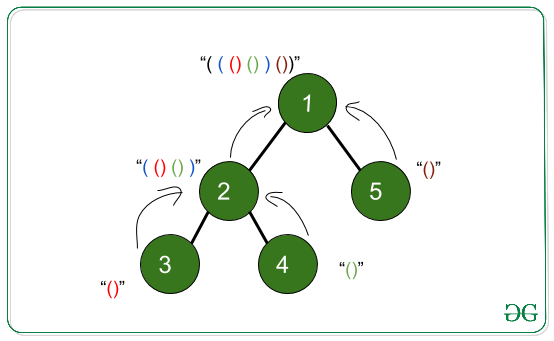# Isomorphism in N-ary Trees

• Difficulty Level : Hard
• Last Updated : 16 Aug, 2021

Given two N-ary trees having M nodes each. Also, given their edges and their roots respectively. The task is to check if they are isomorphic trees or not. If both the trees are isomorphic then print “Yes” else print “No”.
Examples:

Input: M = 9, Root Node of tree-1: 1, Root Node of tree-2: 3
Edges of Tree-1: { (1, 3), (3, 4), (3, 5), (1, 8), (8, 9), (1, 2), (2, 6), (2, 7) }
Edges of Tree-2: { (3, 1), (1, 2), (1, 5), (3, 6), (6, 7), (3, 4), (4, 8), (4, 9) }

Attention reader! Don’t stop learning now. Get hold of all the important DSA concepts with the DSA Self Paced Course at a student-friendly price and become industry ready.  To complete your preparation from learning a language to DS Algo and many more,  please refer Complete Interview Preparation Course.

In case you wish to attend live classes with experts, please refer DSA Live Classes for Working Professionals and Competitive Programming Live for Students.Output: YES
Input: M = 9, Root Node of tree-1: 6, Root Node of tree-2: 7
Edges of Tree-1: {(1, 3),(1, 2), (1, 8), (3, 4), (3, 5), (8, 9), (2, 6), (2, 7)}
Edges of Tree-2: {(1, 2), (1, 5), (3, 1), (3, 4), (4, 8), (4, 9), (6, 3), (7, 6)}Output: NO

Approach:
The idea is to find out the canonical form of both the trees and comparing them. The leaf node will return “()” to its subsequent upper levels.
Below is the example showing the process of finding the canonical form.Below is the implementation of the above approach:

## CPP

 `// C++ program for the above approach``#include ``using` `namespace` `std;` `// To create N-ary tree``map<``int``, vector<``int``> > tree;` `// Function which will accept the root``// of the tree and its parent (which``// is initially "-1") and will return``// the canonical form of the tree``string ConvertCanonical(``int` `vertex,``                        ``int` `parent)``{``    ``// In this string vector we will``    ``// store canonical form of out``    ``// current node and its subtree``    ``vector child;` `    ``// Loop to the neighbours of``    ``// current vertex``    ``for` `(``auto` `neighbour : tree[vertex]) {` `        ``// This is to prevent us from``        ``// visiting the parent again``        ``if` `(neighbour == parent)``            ``continue``;` `        ``// DFS call neighbour of our``        ``// current vertex & it will``        ``// pushback the subtree-structure``        ``// of this neighbour into our``        ``// child vector.``        ``child.push_back(ConvertCanonical(``            ``neighbour, vertex));``    ``}` `    ``// These opening and closing``    ``// brackets are for the``    ``// current node``    ``string str = ``"("``;` `    ``// Sorting function will re-order``    ``// the structure of subtree of``    ``// the current vertex in a``    ``// shortest-subtree-first manner.``    ``// Hence we can``    ``// now compare the two tree``    ``// structures effectively``    ``sort(child.begin(), child.end());` `    ``for` `(``auto` `j : child)``        ``str += j;` `    ``// Append the subtree structure``    ``// and enclose it with "(" ``    ``// ")" the opening and closing``    ``// brackets of current vertex``    ``str += ``")"``;` `    ``// return the subtree-structure``    ``// of our Current vertex``    ``return` `str;``}` `// Function to add edges``void` `addedge(``int` `a, ``int` `b)``{``    ``tree[a].push_back(b);``    ``tree[b].push_back(a);``}` `// Driver code``int` `main()``{``    ``// Given N-ary Tree 1``    ``addedge(1, 3);``    ``addedge(1, 2);``    ``addedge(1, 5);``    ``addedge(3, 4);``    ``addedge(4, 8);``    ``addedge(4, 9);``    ``addedge(3, 6);``    ``addedge(6, 7);` `    ``// Function Call to convert Tree 1``    ``// into canonical with 3 is the root``    ``// and the parent of root be "-1"``    ``string tree1 = ConvertCanonical(3, -1);` `    ``// Clearing our current tree``    ``// before taking input of``    ``// next tree``    ``tree.clear();` `    ``// Given N-ary Tree 2``    ``addedge(1, 3);``    ``addedge(3, 4);``    ``addedge(3, 5);``    ``addedge(1, 8);``    ``addedge(8, 9);``    ``addedge(1, 2);``    ``addedge(2, 6);``    ``addedge(2, 7);` `    ``// Function Call to convert Tree 2``    ``// into canonical``    ``string tree2 = ConvertCanonical(1, -1);` `    ``// Check if canonical form of both``    ``// tree are equal or not``    ``if` `(tree1 == tree2)``        ``cout << ``"YES"` `<< endl;``    ``else``        ``cout << ``"NO"` `<< endl;` `    ``return` `0;``}`
Output:
`YES`

Time Complexity: O(E * log(E)), where E is the number of edges.
Auxiliary Space: O(E)

My Personal Notes arrow_drop_up